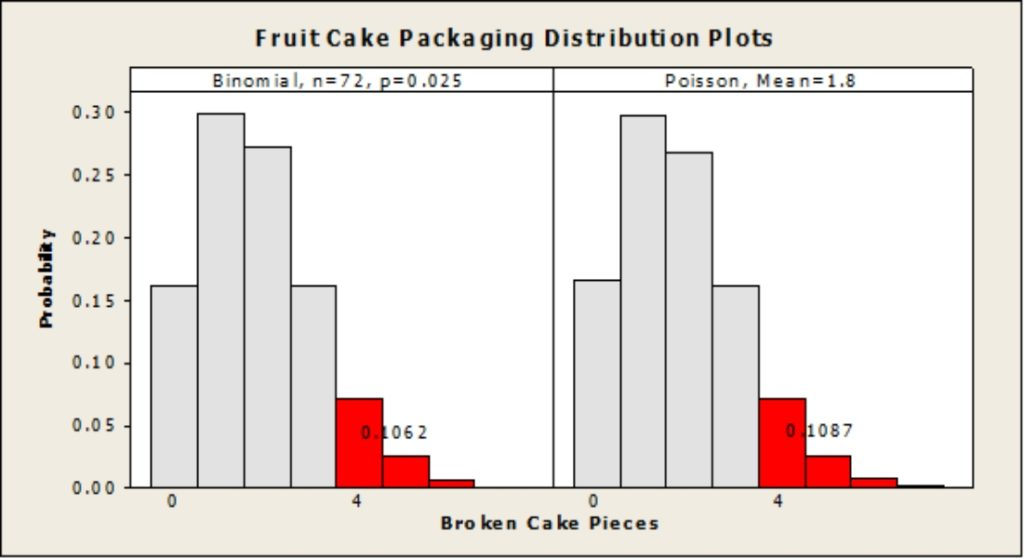# Approximating Binomial Distributions with Poisson DistributionsApproximating Binomial Distributions with Poisson Distributions:

The “law of rare events” or “Poisson limit theorem”, in layman’s terms states that where the independent events have a very low i.e., “rare” probability and number of trials are very large, the Binomial Distributions can be modeled by Poisson Distributions. This was done after Poisson’s work which generalized Bernoulli’s Theorem for n (this is the number of trials) → ∞ (n tends to infinity).

For the Binomial Distributions, the above holds good, even when “n” is sufficiently large (typically n ≥ 30) and, “p” is close to zero (typically p ≤ 0.05), the Binomial distributions can be approximated with the Poisson distributions,

Let’s, look at an example and understand this further via a visual plots comparison of the two distributions: (created using Minitab)

For a bakery producing and packing sliced fruit cakes, the probability of a single piece of broken cake is 0.025 per container (having 12 boxes each, and boxes have 6 fruit-cake slices each) we need to check the probability of 4 or more pieces broken within a container, do we use a Binomial Distribution or a Poisson Distribution ? Let’s find out …

Here, n = 12*6 = 72 (n is quite large), and p = 0.025 (less than 0.05)

The value of λ (event rate) can be calculated as the product of n and p and therefore λ becomes:

λ = 72*0.025 = 1.8

In this case we see that the answer (probability value for 4 or more broken pieces, red-color coded) we get from Binomial distribution is 0.1062 or 10.62% chance of finding 4 or more broken pieces of cake per container,

And, the answer we get from Poisson distribution (plotted on the right) is 0.1087 or 10.87% chance of finding 4 or more broken pieces of cake per container.

The above answers are approximately equal (atleast for most practical cases):This result shows clearly that in practice, we can easily choose Poisson distribution to approximate the Binomial distribution, when we have sufficiently large number of trials (i.e., n is large),  and when the event probability is quite rare. (i.e., p is very small).

Now, all that talk of cakes ,,,, someone is thinking of tea …. Have a nice day and catch you with another simple blog soon 🙂

However, before we conclude this blog, for those interested in more such topics do visit our blog section and also try our 100% Free and 100% Online Lean Six Sigma White Belt Certification